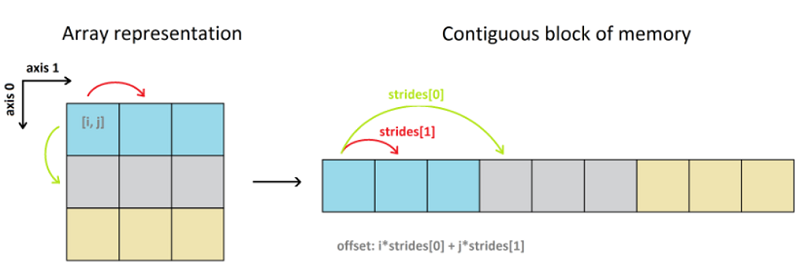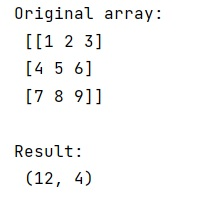# How to understand NumPy strides for layman?

Learn how to understand NumPy strides for layman in Python?
Submitted by Pranit Sharma, on February 11, 2023

NumPy is an abbreviated form of Numerical Python. It is used for different types of scientific operations in python. Numpy is a vast library in python which is used for almost every kind of scientific or mathematical operation. It is itself an array which is a collection of various methods and functions for processing the arrays.

## NumPy strides for layman

There are some homogeneous and contiguous blocks of memory called data buffers which are responsible for storing the actual data of a NumPy array. Using the (default) row-major order, a 2D array looks like this:The strides of an array tell us how many bytes we have to skip in memory to move to the next position along a certain axis. For example, we have to skip 4 bytes (1 value) to move to the next column, but 20 bytes (5 values) to get to the same position in the next row.

If we are given a 3 X 3 numpy array then the number of strides for the horizontal direction (axis-1) is 4 bytes.

Let us understand with the help of an example,

## Python code to demonstrate the example of NumPy strides for layman

```# Import numpy
import numpy as np

# Creating a numpy array
arr =  np.array([[1, 2, 3],[4, 5, 6],[7, 8, 9]])

# Display original array
print("Original array:\n",arr,"\n")

# Getting strides
res = arr.strides

# Display Result
print("Result:\n",res,"\n")
```

Output: